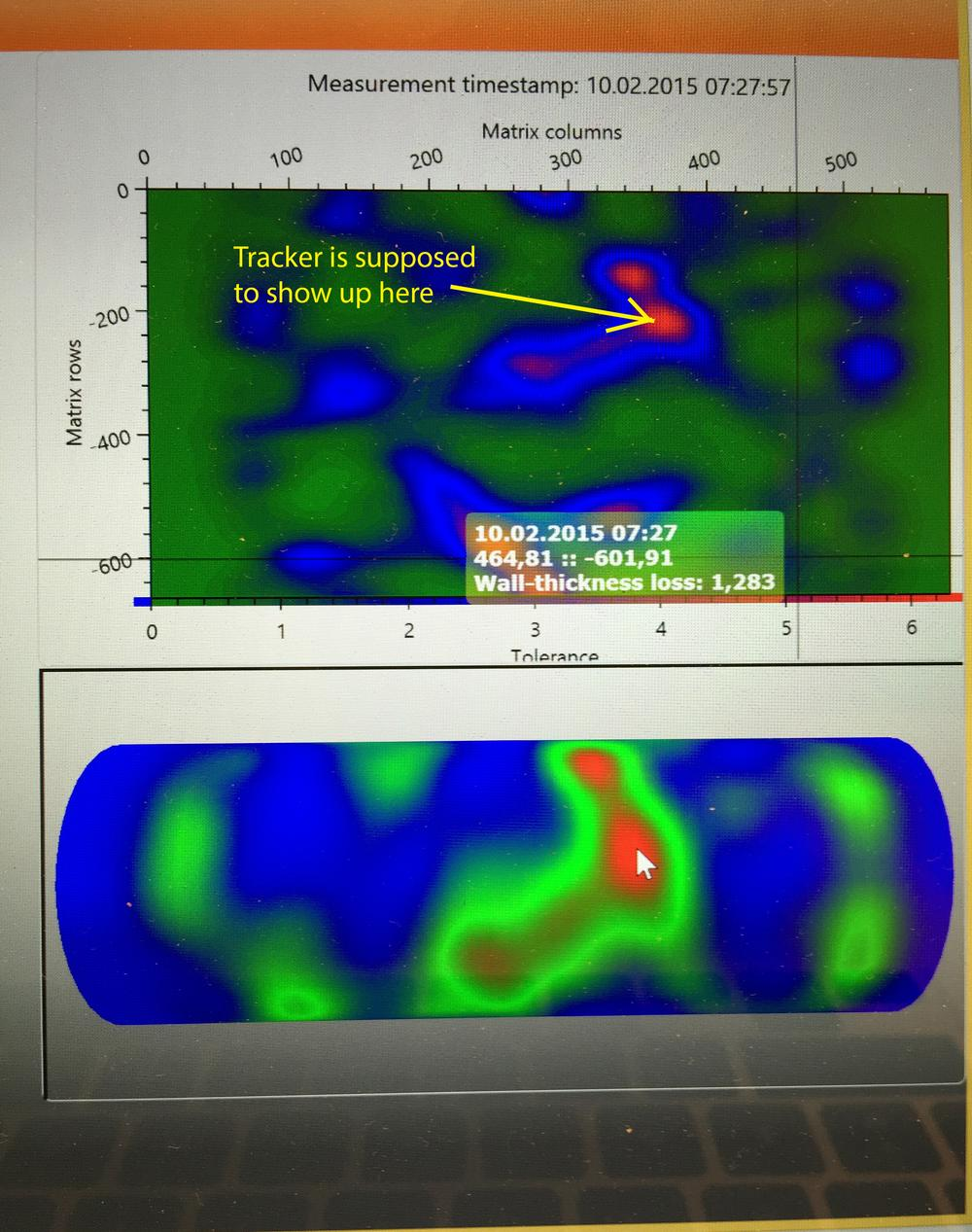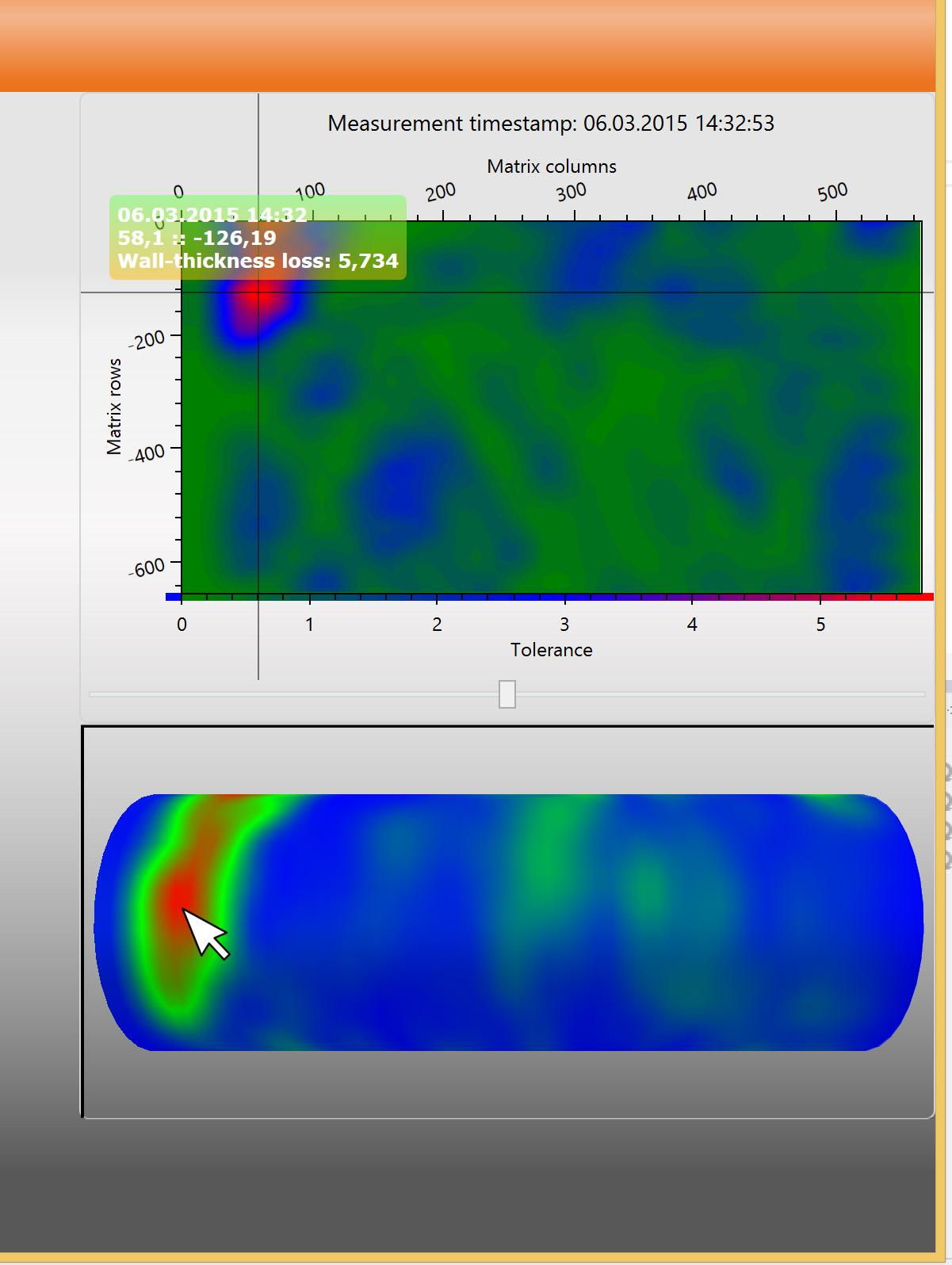0
Under review

# Using TrackerHitResult in OxyPlot.WPF.HeatMapSeries

pettertho89 5 years ago updated by Oystein Bjorke 5 years ago
Hi,

We are able to find this method within oxyplot, but can not find it within oxyplot.wpf.
Is there a reason for this, and how is it possible to use this with a HeatMapSeries in Oxyplot.wpf?Ok, so we have gotten a bit further with this.

To show the tracker in the heatmapseries we need some coordinates. We get these coordinates by clicking a 3D-mesh which gives us the texture coordinates from the model. Using these and our dataset, we can calculate the point which the tracker should be showing in the heatmapseries. However, while we can get the tracker to show, it is off.

As you can see in this picture:This is where the click-event is fired in the ViewModel:
```this.mediator.Register(
param => {
EventHandler<Point> handler = this.ShowTracker;
if (handler != null) {
handler(this, (Point)param);
}
});```

This is the handling of the event in the code behind the user control :
```private void Plot_Loaded(object sender, RoutedEventArgs e) {
(DataContext as CEMC.VM.HeatmapVM).ShowTracker += (s, p) => {
OxyPlot.DataPoint dataPoint = new OxyPlot.DataPoint(p.Y * (hms.X1 - 1), p.X * (hms.Y0 + 1));
OxyPlot.ScreenPoint screenPoint = new OxyPlot.ScreenPoint(dataPoint.X, -(dataPoint.Y));
plot.ShowTracker((hms.InternalSeries as OxyPlot.Series.HeatMapSeries).GetNearestPoint(screenPoint, false));
};
}```

Any help would be gladly appreciated!Hi again, we've made some more progress.

Right now, we have manually coded the offset from the plot to the heatmapseries, and the actual size of the HeatMapSeries itself. This gives us a close result, and is, we suppose, an indication that we are on the right track.
Our problem now, though, is that we don't know how to extract the values automatically from either the Plot or the UserControl.

As you can see, it is getting close:```private void Plot_Loaded(object sender, RoutedEventArgs e) {
//((CEMC.VM.HeatmapVM)DataContext).ShowTracker += this.Tracker;
(DataContext as CEMC.VM.HeatmapVM).ShowTracker += (s, p) => {
OxyPlot.DataPoint dataPoint = new OxyPlot.DataPoint(p.Y * (hms.X1), p.X * (hms.Y0));
OxyPlot.ScreenPoint screenPoint = new OxyPlot.ScreenPoint(dataPoint.X, -(dataPoint.Y));
DataPoint datapoint = new DataPoint(p.Y*466+60, p.X*233+79);
ScreenPoint screenPoint = new Point(datapoint2.X,datapoint2.Y).ToScreenPoint();

plot.ShowTracker((hms.InternalSeries as OxyPlot.Series.HeatMapSeries).GetNearestPoint(screenPoint, false));

};```
p:
This is the parameter that is being sent from the Viewport to the HeatMap and contains TextureCoordinates of the 3D-model.
This gives us coordinates from 0 to 1 in both x and y.
For the picture above, the TextureCoordinates are: X: 0.199695799374776 :: Y: 0.112124022891935

DataPoint:
Where 466 is the width of the heatmapseries, 233 is the height of the heatmapseries. 60 is the offset in width from plot to heatmapseries, and 79 is the offset in height from plot to heatmapseries.

When we try to get Width, or ActualWidth, we can not get a hold of the values. They are either 0 or NaN, as they are not set explicitly.

If I'm right, these values I have used to set width/height and margins will change according to the screen resolution, correct me if I'm wrong.

The question then becomes:
How can we get the values for both width/height of the heatmapseries, and also offset x/y from the plot to the heatmapseries?

As always, any help will be much appreciated, and please ask if anything is unclear.Back again!

After some more work and pondering with this, we've found something that works for us.

To get the Width/Height/etc. of the HeatMapSeries, we had to use the PlotModels PlotArea. This way we can get the correct values to position the tracker correctly and thus, the method does what is it supposed to.

```private void Plot_Loaded(object sender, RoutedEventArgs e) {
(DataContext as CEMC.VM.HeatmapVM).ShowTracker += (s, p) => {
OxyRect plotArea = plot.ActualModel.PlotArea;
ScreenPoint sp = new ScreenPoint(p.Y * plotArea.Width + plotArea.Left, p.X * plotArea.Height + plotArea.Top);
plot.ShowTracker((hms.InternalSeries as OxyPlot.Series.HeatMapSeries).GetNearestPoint(sp, false));
};
}```

Sorry for using this thread as our rubberduck!Under review
No problem! The rubberduck is always very helpful :-)
The OxyPlot.Wpf classes are not covering the full functionality of the classes they are wrapping, I don't think any events are implemented yet. For full functionality I recommend using the PlotView and a PlotModel!